# Sum result is NaN

When I sum TOTAL column, result is Nan
I'm using this section on my code and ı wanna change as float.
@string.Format("{0:N}", item.TOTAL_NET)

How can I fix?
thnx.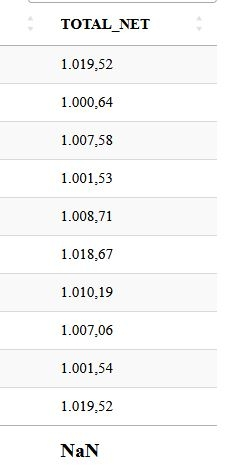I'm using this section for sum.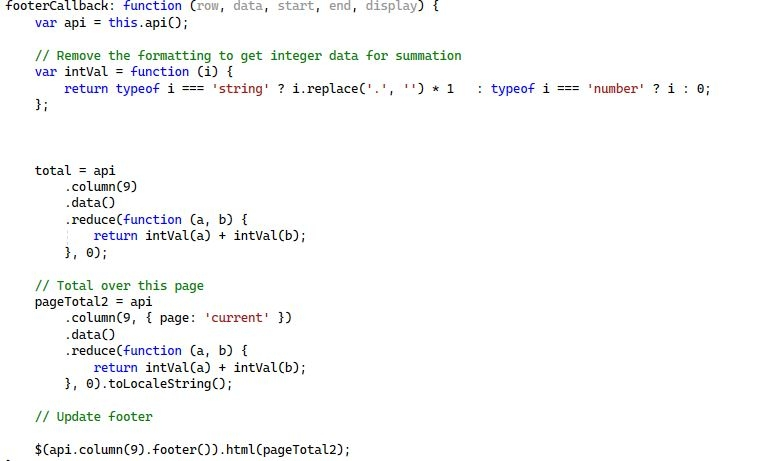You are using non-numeric numbers. Hence it cannot work. The world of software is US dominated. So 1.019,52 is just some string, but not a number. 1,019.52 would be a proper number. And 1019.52 too.

In your intVal function (which I would call floatVal by the way) you are replacing periods with spaces. So you get 1019,52 which is not a number. You need to replace
1. the periods with spaces and then
2. the remaining comma with a period

``````return typeof i === 'string' ? i.replace(/[\.]/g, '').replace(/[\,]/g, '.') * 1 : typeof i === 'number' ? i : 0;
``````

P.S.: I didn't check the rest of your code. Just give it a try first.

• edited November 2022

This code call data as string. But before I tried parse to float, I need to replace.
Like that.

``````                total = api
.column(9)
.data()
.reduce(function (a, b) {
a=a.replace('.','');
a=parseFloat(a);
b=b.replace('.','');
b=parseFloat(b);
return intVal(a) + intVal(b);
}, 0);
``````

But this is not working. What should I do?

edited November 2022

Not sure I understand what you are talking about ... This code should give you the footer with the total in the same formatting as your input numbers.

``````footerCallback: function (row, data, start, end, display) {
var api = this.api();
var numberRenderer = \$.fn.dataTable.render.number( '.', ',', 2 ).display;

// Remove the formatting to get float data for summation
var floatVal = function (i) {
return typeof i === 'string' ? i.replace(/[\.]/g, '').replace(/[\,]/g, '.') * 1 : typeof i === 'number' ? i : 0;
};

// Total over current page
var total = api
.column( 9, { page: 'current' } )
.data()
.reduce(function (a, b) {
return floatVal(a) + floatVal(b);
}, 0);
// Update footer
\$( api.column(9).footer() ).html( numberRenderer(total) );
}
``````

This would be the result. The green line is the footer.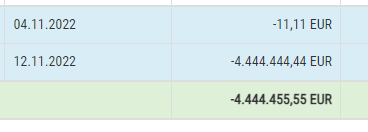The full code for the example above is this. It is flexible regarding the user language (English and German; German being the same format as yours)

``````footerCallback: function (row, data, start, end, display) {
var api = this.api();
if (lang == 'de') {
var numberRenderer = \$.fn.dataTable.render.number( '.', ',', 2 ).display;
} else {
var numberRenderer = \$.fn.dataTable.render.number( ',', '.', 2 ).display;
}

// Remove the formatting to get integer data for summation
var floatVal = function (i) {
if (lang == 'de') {
return typeof i === 'string' ? i.replace(/[\.]/g, '').replace(/[\,]/g, '.') * 1 : typeof i === 'number' ? i : 0;
} else {
return typeof i === 'string' ? i.replace(/[\,]/g, '') * 1 : typeof i === 'number' ? i : 0;
}
};

// Total over all pages
var twoDecPlacesCols = [3, 6];
var total;
var curr = data.ctr.currency;
for ( i=0; i < twoDecPlacesCols.length; i++ ) {
total = api
.column(twoDecPlacesCols[i])
.data()
.reduce(function (a, b) {
return floatVal(a) + floatVal(b);
}, 0);
// Update footer
\$(api.column(twoDecPlacesCols[i]).footer()).html( numberRenderer(total) + " " + curr );
}
}
``````
• When I use your code, nothing is returning on sum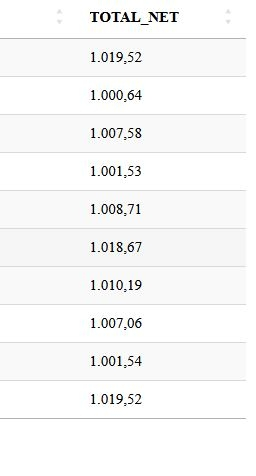... Then you have a mistake somewhere ...

Please post a test case as per the forum rules. Thanks.

• WEll, I call to Total_NET as a string, it's not numeric. But before I sum them, I need to convert as numeric.
I can use `\$.fn.dataTable.render.number('.', ',', 2, '')` But when I use that,it doesn't seen after the ' , '

WEll, I call to Total_NET as a string

What does that mean? I don't understand it..

If you call the number renderer with something in the format 9999.99 it should work. And yes the number renderer does not return a number because a formatted number 9.999,99 is not a number. Hence it's gotta be a string.

In your debugger please check the format of "total". If it is not 9999.99 you have a mistake somewhere.

• if i change the question, I called that column as numeric and sum is correct. But I see '##,00'.
How can I fix 00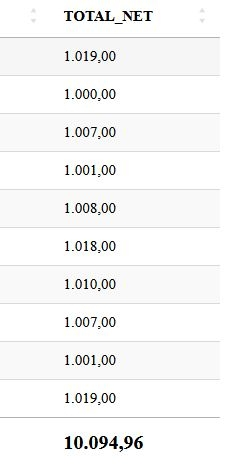• It's code

``````    \$(document).ready(function () {
\$('#example').DataTable({
dom: 'lBfrtip',
scrollX: true,

footerCallback: function (row, data, start, end, display) {
var api = this.api();
var numberRenderer = \$.fn.dataTable.render.number('.', ',', 2).display;

// Remove the formatting to get float data for summation
var floatVal = function (i) {
return typeof i === 'string' ? i.replace(/[\.]/g, '').replace(/[\,]/g, '.') * 1 : typeof i === 'number' ? i : 0;
};

// Total over current page
var total = api
.column(9, { page: 'current' })
.data()
.reduce(function (a, b) {
return floatVal(a) + floatVal(b);
}, 0);
// Update footer
\$(api.column(9).footer()).html(numberRenderer(total));
}
});
});
``````

But I see '##,00'.
How can I fix 00

What does that mean?
To me your pic looks good.

Don't seem to understand you very well. Sorry about that.

• for example first row should be 1.019,52 but it seen 1.019,00